Reach Us+44-7482-875032
Fractal Functions in Interpolation and Approximation: A Bird's-Eye View | OMICS International
Journal of Applied & Computational Mathematics
All submissions of the EM system will be redirected to Online Manuscript Submission System. Authors are requested to submit articles directly to Online Manuscript Submission System of respective journal.

# Fractal Functions in Interpolation and Approximation: A Bird's-Eye View

Navascues MA1*, Chand AKB2, Viswanathan P3 and Sebastan MV4

1Departmento de Matem´atica Aplicada, Escuela de Ingenier´ia y Arquitectura, Universidad de Zaragoza, Spain

2Department of Mathematics, Indian Institute of Technology Madras, Chennai-600036, India

3Mathematical Sciences Institute, Australian National University, Australia

4Centro Universitario de la Defensa de Zaragoza Academia General Militar, Spain

*Corresponding Author:
Navascues MA
Departmento de Matem´atica Aplicada
Escuela de Ingenier´ia y Arquitectura
Universidad de Zaragoza, Spain
Tel: 34976761000
E-mail: [email protected]

Received Date: October 24, 2014; Accepted Date: October 25, 2014; Published Date: October 31, 2014

Citation: Navascues MA, Chand AKB, Viswanathan P, Sebastan MV (2014) Fractal Functions in Interpolation and Approximation: A Bird's-Eye View. J Appl Computat Math 3:188. doi: 10.4172/2168-9679.1000188

Copyright: © 2014 Navascues MA, et al. This is an open-access article distributed under the terms of the Creative Commons Attribution License, which permits unrestricted use, distribution, and reproduction in any medium, provided theriginal author and source are credited.

Visit for more related articles at Journal of Applied & Computational Mathematics

#### Prologue

Construction of a continuous function from given discrete data, which is referred to as interpolation, has been applied in various fields since time immemorial. In classical Numerical Analysis and Approximation Theory, there are several interpolation methods (for instance, polynomial, trigonometric, spline, rational) that can be applied to a specific data set, according to the assumptions that underlie the model to be investigated. Undoubtedly, these traditional (non-fractal) nonrecursive techniques constitute a great tool to model various physical phenomena. However, these methods produce interpolants with Hausdorff dimension one and hence if a given data set is more complex and irregular (for instance, data sampled from real-world signals such as financial series, seismic data, and bioelectric recordings), then they may not provide satisfactory results. Consequently, to model these signals, need for interpolants that are nondifferentiable in dense set of points in the domain arose quite naturally. About a quarter century has passed since Barnsley  introduced Fractal Interpolation Function (FIF), a concept which soon turned out to be the right frame for a constructive approximation theory of non-differentiable functions, by using the notion of Iterated Function System (IFS). In view of their diverse applications, there has been steadily increasing interest in the theory of fractal functions, and it still continues to be a hot topic of research. Following the publication of Fractals Everywhere , a beautiful exposition of IFS theory, fractal functions and their applications, various related issues such as calculus, Holder continuity, convergence, stability, smoothness, determination of scaling parameters, and perturbation error have been investigated in the literature [3-13]. The concept of smooth FIFs has been used to generalize the traditional splines [14-18] and to demonstrate that the interaction of classical numerical methods with fractal theory provides new interpolation schemes that supplement the existing ones. Various other extensions of FIFs include multivariable FIFs [7,9,16,19-25] generated by using higher-dimensional or recurrent IFSs, the hidden variable FIFs produced by projecting the attractors of vector valued IFSs to a lower-dimensional space.

The notion of FIFs can be used to associate a family of fractal functions fα parameterized by a suitable vector α with a prescribed continuous function f on a compact interval I. Using this “fractal perturbation”, Navascues introduced the operator Fα : c(I)→c(I) via Fα(f) = fα and its extension to more general spaces Lp(I) using standard density arguments. These maps tend to bridge the gap between smoothness of classical mathematical objets and pseudo-randomness of experimental variables, breaking in this way their apparent diversity. Navascues and coworkers [17,18,22] contributed to the theory by defining ”rough” approximants as perturbation of the functions generally used in classical approximation (polynomial, trigonometric, rational, etc.) via this operator. Recently , the authors investigated conditions on the elements of the IFS so that the perturbation preserves the basic shape properties inherent in the original function, thereby paving a way to shape preserving fractal approximation, an interesting area that needs further exploration at least in our opinion.

#### A Brief Outline of Fractal Interpolation Function

By fractal function, we mean basically a function whose graph is the attractor of an IFS, which was first constructed as an interpolant as follows. Consider a set of data points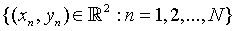with increasing abscissae. Set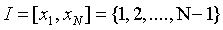. For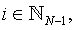set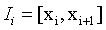and let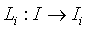be affine maps such that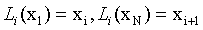Let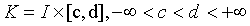Consider N − 1 continuous maps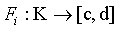satisfying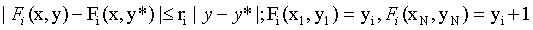(1)

Where x∈I, y, y*∈[c,d] , and 0 ≤ ri < 1. Now define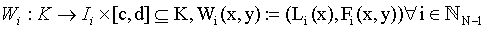For the collection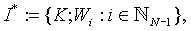which is termed IFS, Barnsley presented the following seminal result.

Theorem 1 (Barnsley ) Corresponding to the IFS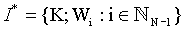there exists a unique set G satisfying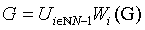and G is the graph of a continuous function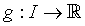satisfying g(xn)=yn for n=1, 2,…N. Let

Let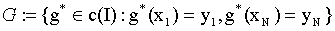be endowed with the uniform metric. If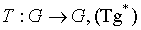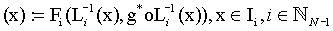then g is the unique xed point of T.

Definition 1 The function g appearing in the previous theorem is called a Fractal Interpolation

Function (FIF) and it fulfills the functional equation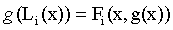for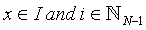Most widely studied fractal functions in the theory and applications heretofore are defined by the

IFS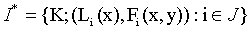with constituent maps(2)

Where |αi| < 1 and qi are suitable continuous functions so that the maps Fi satisfy conditions in (1). A possible explanation for the choice of this special class of IFS is that the corresponding FIF is explicitly integrable, a satisfactory theory for moment integrals can be developed and it is easy to discover functional equations obeyed by Fourier and Laplace transforms. Following Theorem 1, the FIF g can be constructed recursively using the functional equation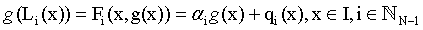(3)

In what follows, we shall get into some specifics of particular flavors of fractal interpolation for the benefit of the reader, specifically for one who wants to critically compare the FIF scheme with schemes available in the traditional Numerical Analysis. It is plain to see that the presence of free parameters i, which determine Hausdorff dimension of the graph of the interpolant, provides additional flexibility to the choice of particular function in contrast to the unicity inherent in a specific kind of traditional interpolation. However, finding an “optimal” scale vector is a quite challenging problem for which different approaches are discussed in the literature. Note that apart from this recursive functional equation, the FIF g possesses an explicit representation in terms of an infinite series . Further, g can be expressed using the technique of operator approximation, see . As with wavelets and many other new function types, “closed form” expressions for FIF generally take the form of one of the two types of algorithms, chaos game (a Markov chain Monte Carlo algorithm) and deterministic iteration; both approaches are highly accurate and have been reported in many places in the literature [5,6]. In many cases, evaluation of a FIF at a specific point can be achieved by summing a rapidly convergent series. The functional equation for the FIF provides a rule to predict the values of the interplant at refined mesh points, and thus may remind a subdivision scheme available in the traditional Numerical Analysis. Let us note here that a FIF, in fact, provides a subdivision scheme, and the fact that it arises from an IFS makes the mathematical treatments such as convergence, smoothness, etc. of those subdivision schemes relatively easier to handle. Most of the traditional interpolants have locality, whereas particular feature of the fractal methods is that they explore the self-similarity and are global, in general. The benefit is that subtle global dependencies reflect nature and may lead to harmonious and attractive non-cookie cutter forms, shapes and approximants. The same is true for Bezier curves, much used in computer graphics and design packages. It should be borne in mind that for particular values of the parameters, the fractal interpolation schemes can recapture local traditional schemes.

#### Fractal Functions in Approximation and Fractal Operator

Reviving the spirit of the previous section, let f ∈ c(I) Consider (2) with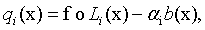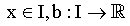is a continuous function, b(x1)=f(x1), b(xN)= f(xN), and b ≠ f. In view of (3), corresponding fractal function fα satisfies.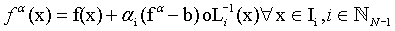(4)

Notice that if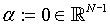then fα = f and also that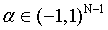is arbitrary. Consequently, (4) associates a family of continuous functions with each fixed f ∈c(I) . The degrees of freedom offered by this procedure may be useful when some problems combined with approximation and optimizations have to be approached. Assume that the continuous function b depends linearly on f, say for instance, b=Lf, where L : c(I)→c(I) is a linear and bounded operator with respect to the uniform norm or Lp norm on C(I). Then the map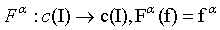defines a linear operator referred to as an α -fractal operator. For various properties of this map, its extension to Lp spaces, and related developments, the reader is urged to refer researches f Navascu´es and coworkers scattered across the literature. However, here let us note the following: (i) the diversity option obtained due to the presence of parameters can be explored to choose best in a problem of approximation combined with optimization, (ii) the perturbation methodology described may serve to modify or preserve the properties of f, for instance, to reduce the order of regularity of the classical approximant, (iii) the procedure provides new geometric possibilities, that is, in the non-smooth case, the graph owns a fractal dimension and this parameter provides an index for experimental signals.

Let us conclude this short article by hinting at the applications of fractal functions. Having provided the above brief introduction to fractal interpolation and its interconnection with classical approximants, it is not difficult to find applications of FIFs in almost every field wherein information available in finite number of grid points has to be modeled with a continuous function. The realm of applications includes geometric design, data visualization, reverse engineering, physics and chemistry, image compression, signal processing, and wavelet theory. The reason for this variety of applications lies in the underlying complicated mathematical structure of fractal functions, produced with simple recursive construction. It has been noted that for certain problems, they provide better approximants than their classical nonrecursive counterparts. For a specific application, let us mention that in reference , fractal interpolation of electroencephalographic recordings is used to describe the increase in the bioelectric complexity during some tests of attention in children and to compute other electroencephalographic parameters. Many areas of fractal functions and their applications are yet to be explored, for instance, calculating Hausdorff dimension of a general FIF is a challenging open problem.

We strongly believe that the field of fractal functions has bright future, however to be able to contribute to it, the reader should leave the idea of staying in the comfort of well-known classical approximation theory and start enjoying the benefits of the more versatile fractal approximants, to supplement the former if not to replace.

#### References

Select your language of interest to view the total content in your interested language

### Article Usage

• Total views: 13192
• [From(publication date):
December-2014 - Aug 26, 2019]
• Breakdown by view type
• HTML page views : 9329
• PDF downloads : 3863

## Post your commentCan't read the image? click here to refresh
###### Peer Reviewed Journals

Make the best use of Scientific Research and information from our 700 + peer reviewed, Open Access Journals

International Conferences 2019-20

Meet Inspiring Speakers and Experts at our 3000+ Global Annual Meetings

Top